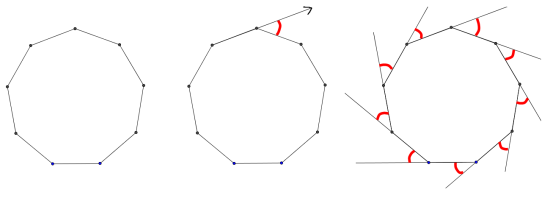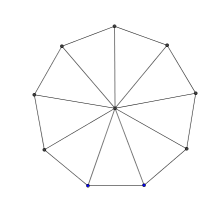#### You may also like### On Time

On a clock the three hands - the second, minute and hour hands - are on the same axis. How often in a 24 hour day will the second hand be parallel to either of the two other hands?### Estimating Angles

How good are you at estimating angles?### LOGO Challenge 1 - Star Square

Can you use LOGO to create this star pattern made from squares. Only basic LOGO knowledge needed.

# Shogi Shapes

##### Age 11 to 14Challenge Level

Here are a couple of methods you could use to work out the interior angles of a regular nine-sided polygon (a nonagon):

1.Imagine taking a walk around a regular nine-sided park.
The second picture shows the angle you need to turn through when you reach the first corner.
The third picture shows all the angles you turn through when you walk once round the park.
What angle do you turn through altogether?
What must each exterior angle (the red angles) be?
What must each interior angle be?2. Here is a regular nonagon:

It has been divided into nine isosceles triangles.
What is the total of all the angles in all the triangles?

Now subtract the angles at the centre of the nonagon to work out the total of all the interior angles of the nonagon.

Can you adapt these methods for any regular polygon?